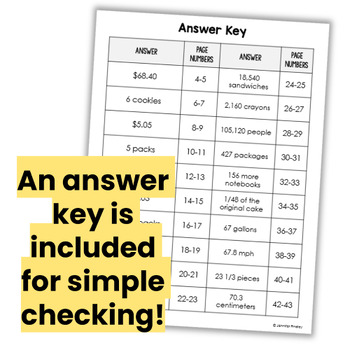# Multi-Step Word Problem Interactive NotebookSubject
Resource Type
Format
PDF (6 MB|20 +Examples)
Standards
\$3.00
\$3.00

#### Also included in

1. This BUNDLE includes 5 of my word problems for interactive notebooks for 5th grade math.This huge bundle includes a total of 137 Word Problems..all the word problems you could need! And the students will love the novel format and engaging clipart that relates to the word problem. These word problems
\$14.00
\$21.00
Save \$7.00

### Description

Multi-Step Word Problems ready to print and use! These word problems include practice with whole numbers, decimals, and fractions (unlike denominators). A skills alignment page is included so you can choose the problems to match the skills you have taught.

Word problems are huge in Common Core and students need to be prepared to not only solve them, but to show and prove their thinking. I created this resource to help my students meet this requirement.

This resource includes 20 multi-step word problems (2 to a page). Depending on the size of the notebook, you may have to print the pages at 80% to have them fit on the page. I printed at 80% to fit them in a spiral notebook.

The students write the solution on the word problem paper and then show their work, explain the steps they took or prove their answer underneath the word problem on their notebook paper.

Each word problem comes with a sample completed word problem showing the steps to solve each one. The first four include the steps summarized to have an example for your students.

I have included an answer key and skills alignment. This resource includes operations with fractions, decimals, and whole numbers.

You may also like:
Interactive Math Notebook: Word Problems {5th Grade: Common Core Aligned}Interactive Math Notebook: Word Problems {4th Grade: Common Core Aligned}

For affordable, no-fuss flippables (perfect for beginning interactive notebook users) click on the links below.
Math Interactive Student Notebook: Flippables {5th Grade: Common Core Aligned}Math Interactive Student Notebook: Flippables {4th Grade: Common Core Aligned}
Total Pages
20 +Examples
Included
Teaching Duration
2 months
Report this Resource to TpT
Reported resources will be reviewed by our team. Report this resource to let us know if this resource violates TpT’s content guidelines.

### Standards

to see state-specific standards (only available in the US).
Solve real world problems involving multiplication of fractions and mixed numbers, e.g., by using visual fraction models or equations to represent the problem.
Apply and extend previous understandings of multiplication to multiply a fraction or whole number by a fraction.
Add and subtract fractions with unlike denominators (including mixed numbers) by replacing given fractions with equivalent fractions in such a way as to produce an equivalent sum or difference of fractions with like denominators. For example, 2/3 + 5/4 = 8/12 + 15/12 = 23/12. (In general, 𝘢/𝘣 + 𝘤/𝘥 = (𝘢𝘥 + 𝘣𝘤)/𝘣𝘥.)
Add, subtract, multiply, and divide decimals to hundredths, using concrete models or drawings and strategies based on place value, properties of operations, and/or the relationship between addition and subtraction; relate the strategy to a written method and explain the reasoning used.
Find whole-number quotients of whole numbers with up to four-digit dividends and two-digit divisors, using strategies based on place value, the properties of operations, and/or the relationship between multiplication and division. Illustrate and explain the calculation by using equations, rectangular arrays, and/or area models.